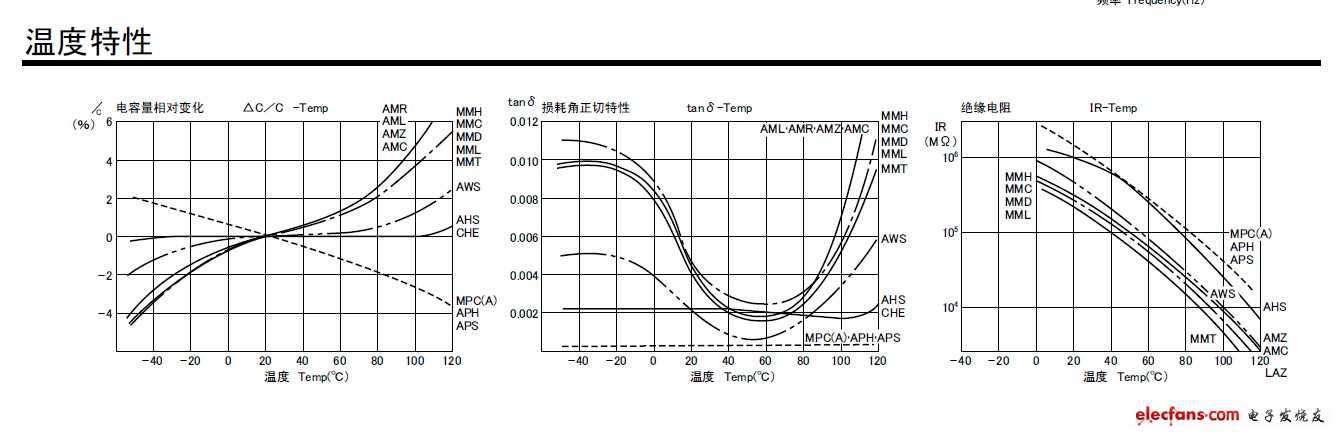|
|
» » » What is the temperature characteristic of the capacitor

# What is the temperature characteristic of the capacitor

Views:3     Author:Site Editor     Publish Time: 2020-06-19      Origin:Site

When the temperature of the capacitor becomes higher, the capacitance can become lower or higher.1. Temperature and capacitor life. In general, the life of capacitors decreases with increasing temperature, the most obvious of which is electrolytic capacitors. Electrolytic capacitors with a limit operating temperature of 85°C, working at a temperature of 20°C, generally guarantee 18,019 hours of normal working time; Working under the limit temperature of 85 , generally can only guarantee the normal working time of 2000 hours. Therefore, you should pay attention to this situation when designing the circuit.

2. The loss tangent of temperature and capacitance. Any capacitor has a loss tangent value, which is the loss of the capacitor. The tangent value generally increases with increasing temperature. For example, the loss angle tangent of the CC10 type UHF ceramic dielectric capacitor is 0.0012 at normal temperature (20℃±5), and is 0.0018 at positive limit temperature (85). It can be seen that it is greatly affected by temperature.

3. Insulation resistance of temperature and capacitance. Generally, the insulation resistance of a capacitor decreases with increasing temperature, and the decrease in insulation resistance causes the leakage current of the capacitor to increase.

4. Temperature and capacitance. The temperature coefficient of a capacitor means that the capacity of the capacitor changes with temperature. When designing a precision capacitor timing circuit and an oscillation circuit whose frequency is determined by the capacitor, the influence of temperature on the capacitor capacity should be fully considered. Otherwise, the designed capacitor timing circuit will be inaccurate; the oscillation frequency of the oscillation circuit will change with temperature.

In general, when using capacitors, the influence of temperature on the capacitor should be fully considered, and the capacitor should be operated at about 20°C as much as possible to avoid the influence of temperature on many parameters of the capacitor.

Capacitor temperature coefficient Capacitor temperature coefficient is divided into level I and level II. Class I capacitors are divided into 8 classes, and class II capacitors are divided into 5 classes.